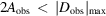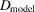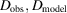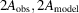International
Tables for
Crystallography
Volume A
Space-group symmetry
Edited by M. I. Aroyo

International Tables for Crystallography (2016). Vol. A, ch. 1.6, p. 126

## Section 1.6.5.1.4. Data evaluation after structure refinement

H. D. Flackb

#### 1.6.5.1.4. Data evaluation after structure refinement

| top | pdf |

There is an excellent way in which to evaluate both data measurement and treatment procedures, and the fit of the model to the data, including the space-group assignment, at the completion of structure refinement. This technique is applicable both to noncentrosymmetric and to centrosymmetric crystals. A scattergram of Dobs against Dmodel, and 2Aobs against 2Amodel pairs are plotted on the same graph. All (Dobs, Dmodel) pairs are plotted together with those (2Aobs, 2Amodel) pairs which have. The range of values on the axes of the model and of the observed values should be identical. For acentric reflections, for both A and D, a good fit of the observed to the model quantities shows itself as a straight line of slope 1 passing through the origin, with some scatter about this ideal straight line. For an individual reflection, 2A and D are, respectively, the sum and the difference of the same quantities and they have identical standard uncertainties. It is thus natural to select 2A and D to plot on the same graph. In practice one sees that the spread of the 2A plot increases with increasing value of 2A. Fig. 1.6.5.1shows the 2AD plot for Ex2 of Example 2 in Section 1.6.5.1.2, which is most satisfactory and confirms the choice of point group from the use of Rmerge. The conventional R value for all reflections is 3.1% and for those shown in Fig. 1.6.5.1it is 10.4%. The R value for all D values is good at 51.1%. Fig. 1.6.5.2shows the 2AD plot for Ex1 of Example 1 in Section 1.6.5.1.2. The structure model is centrosymmetric so allvalues are zero. The conventional R value on A for all reflections is 4.3% and for those shown in Fig. 1.6.5.2it is 9.1%. The R value on all the D values is 100%.Figure 1.6.5.1 | top | pdf |Data-evaluation plot for crystal Ex2. The plot shows a scattergram of all () pairs and those () pairs in the same intensity range as the D values.Figure 1.6.5.2 | top | pdf |Data-evaluation plot for crystal Ex1. The plot shows a scattergram of all () and some () data points.